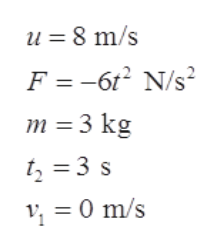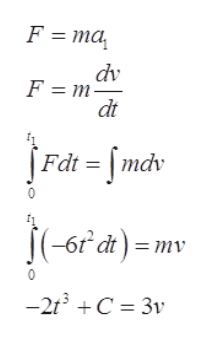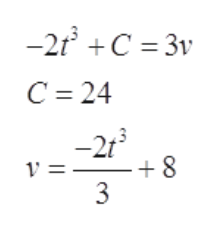A 3.00 kg box is moving to the right with speed 8.00 m/s on a horizontal, frictionless surface. At t = 0 a horizontal force is applied to the box. The force is directed to the left and has magnitude F(t)=( 6.00 N/s2 )t2What distance does the box move from its position at t=0 before its speed is reduced to zero?If the force continues to be applied, what is the velocity of the box at 3.00 s ?

Question

A 3.00 kg box is moving to the right with speed 8.00 m/s on a horizontal, frictionless surface. At t = 0 a horizontal force is applied to the box. The force is directed to the left and has magnitude F(t)=( 6.00 N/s2 )t2

What distance does the box move from its position at t=0 before its speed is reduced to zero?
If the force continues to be applied, what is the velocity of the box at 3.00 s ?
Step 1

Let us assume the box is initially moving with a velocity u, when the force is applied then the box is subjected to constant decrement in the velocity. It takes time t1 to reduce the velocity v1 to zero, let s1 be the distance travelled by the box in t1 time. In time t2 assume the velocity of the box is v2.

As the motion is in horizontal plane consider right direction to be positive and left direction to be negative.help_outlineImage Transcriptioncloseu = 8 m/s F -6 N/s m 3 kg t23 s 14 0 m/s fullscreen
Step 2

Write the expression for Newton’s second law, and substitute the above found values.help_outlineImage TranscriptioncloseF ma dv F m- dt [Fât = [mdv (-6 dt=mv -2t3 C 3v fullscreen
Step 3

To find the constant C. put the ini...help_outlineImage Transcriptionclose-2 C 3v C 24 8 3 en fullscreen

Want to see the full answer?

See Solution

Want to see this answer and more?

Our solutions are written by experts, many with advanced degrees, and available 24/7

See Solution
Tagged in

Physics